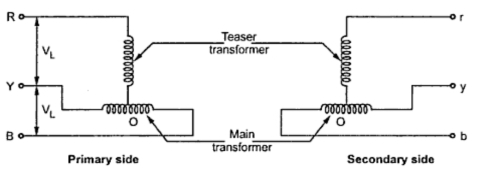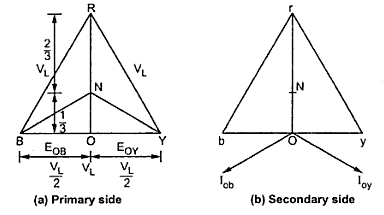### The T-T connection for 3-Phase Transformer

This type of connection uses tow transformers as that open delta connection. With the help of this type of connection the conversion from 3-phase is possible which is shown in the Fig. 1.Fig.  1
This connection was proposed by Charles F Scott connection. The conversion of  3-phase to 2-phase is also accomplished using this connection.
The Fig.2 shows the details of T -T type of connection. One of the transformers in the connection is called main transformer which is proved with a 50% taping on both primary and secondary windings. The other transformer known as teaser transformer having 0.866 tap connected to centre tap on main transformer on primary and secondary side.Fig.  2
The teaser transformer may be designed for 86.6 percent of the line or main transformer voltage but generally is made identical with the main transformer and operated at reduced flux density. Thus it possible to operate tow identical single phase transformer either in T - T or in open delta (V-V) if one of the transformers in a delta-delta bank become inoperative. The only thing is that there should be 50 % tap on one of the transformers.Fig.   3
The phasor diagram indicating the different voltages is shown in the Fig. 3
From the phasor diagram drawn in the primary side it can be seen that EOY and EOB are each (VL/2 ) and differ in phase by 180° as both the parts of the windings on the main transformer are one same magnetic circuit and are connected in opposition. All the sides of the equilateral triangle represents the line voltage VL. The voltage EOR is the altitude of the equilateral and it will be equal to (√3/2) times the line voltage and lags behind the voltage across the main transformer 90°. The same relation can be seen in the secondary winding with r - y- b forming a symmetrical system.
The phasor diagram on secondary side is shown in the Fig.3(b). If we consider the load with unity power factor, current Ioy lags behind voltage Eoy by 30° and current Iob leads Eob by 30°. Thus the teaser transformer and each half of the main transformer all operate at different power factors.
The bank capacity of the T - T  connection is same as for tow transformers connected in open delta. The teaser transformer operate at 0.866 if its rated voltage and the main transformer is operating at the p.f. of cos 30° = 0.866 which is same as the main transformer working at 86.6 % of their KVA rating.
The ratio of KVA utilized to that available would be 0.928 which will make the connection more economical that open ∆. This can be obtained as follows.
Available VA capacity = VL IL + (0.866VL ) IL = 1.866 VL IL
VA actually utilized = 1.732 VL IL since 3-phase power is supplied
Taking the ratio of actual capacity to available capacity
Actual capacity/Available capacity = (1.732 VL IL )/(1.866 VL IL ) = 0.928
For a load of unity p.f. the current in teaser transformer is in phase with the voltage. In the main transformer the current leads voltage in one half by 30° and lags voltage in other half by 30°.
With a load of p.f. cosΦ, the current in teaser transformer will be lag or lead the voltage by Φ while in the tow halves of the main transformer the angle between the current and the voltage will be (30 - Φ) and (30 - Φ) which is same as that in V- V connection.

Related articles :